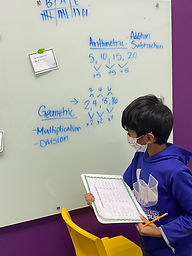## Ms. Briana

### Target 1​

###### Lesson Type:

New

Number Operation

:

Operational Relationships

Use properties of operations as strategies for solving arithmetic problems.

###### 1:

Identify the Commutative Property of Multiplication.

###### 2:

Understand that changing the order of factors, does not change the product.

###### 3:

Understand that multiplication and addition are commutative.

###### 4:

Understand that division and subtraction are not commutative.

3rd

###### Vocabulary:

Cummutative Property, Factors, Product

Activities:

Students attempted switching the order of different operational equations to see if they maintained the same answer.

Students then determined addition and multilication are Commutative but division and subtraction are not.

Students played a commutative matching game to reinforce the concept.### Home Exploration

###### Guiding Questions:## Absent Students:

Nova

### Target 2

:

###### 2:

Create subtraction sequences.

###### 3:

Create multiplication sequences.

###### 4:

Create division sequences.

###### 5:

Use data tables to determine the rule for an arithmetic sequence.

4th

###### Vocabulary:

Sequence, Arithmetric, Geometric

Activities:

Students reviewed arithmetric and geometric Sequence descriptions.

Students then played a game where they used task cards with differenct sequences and rules on them. Based on the information given students had to complete the sewuence and setermine if it was geometric or arithmetic and explain why.### Home Exploration

###### Guiding Questions:### Target 3

:

###### Vocabulary:

Activities:### Home Exploration